# RD Sharma Solutions for Class 11 Chapter 23 - The Straight Lines Exercise 23.9

In Exercise 23.9, we shall discuss the transformation of general equations in different standard forms, with suitable examples for students to understand the concepts clearly. By following the latest Syllabus, the RD Sharma Class 11 Maths Solutions are created and it is framed in accordance with the exam pattern of the CBSE Board. Experts have designed these solutions, assembling model questions covering all the exercise questions from the textbook. The solutions pdf for this exercise can be easily downloaded from the links given below and can be used for future reference, as well.

## Download the pdf of RD Sharma Solutions for Class 11 Maths Exercise 23.9 Chapter 23 – The Straight Lines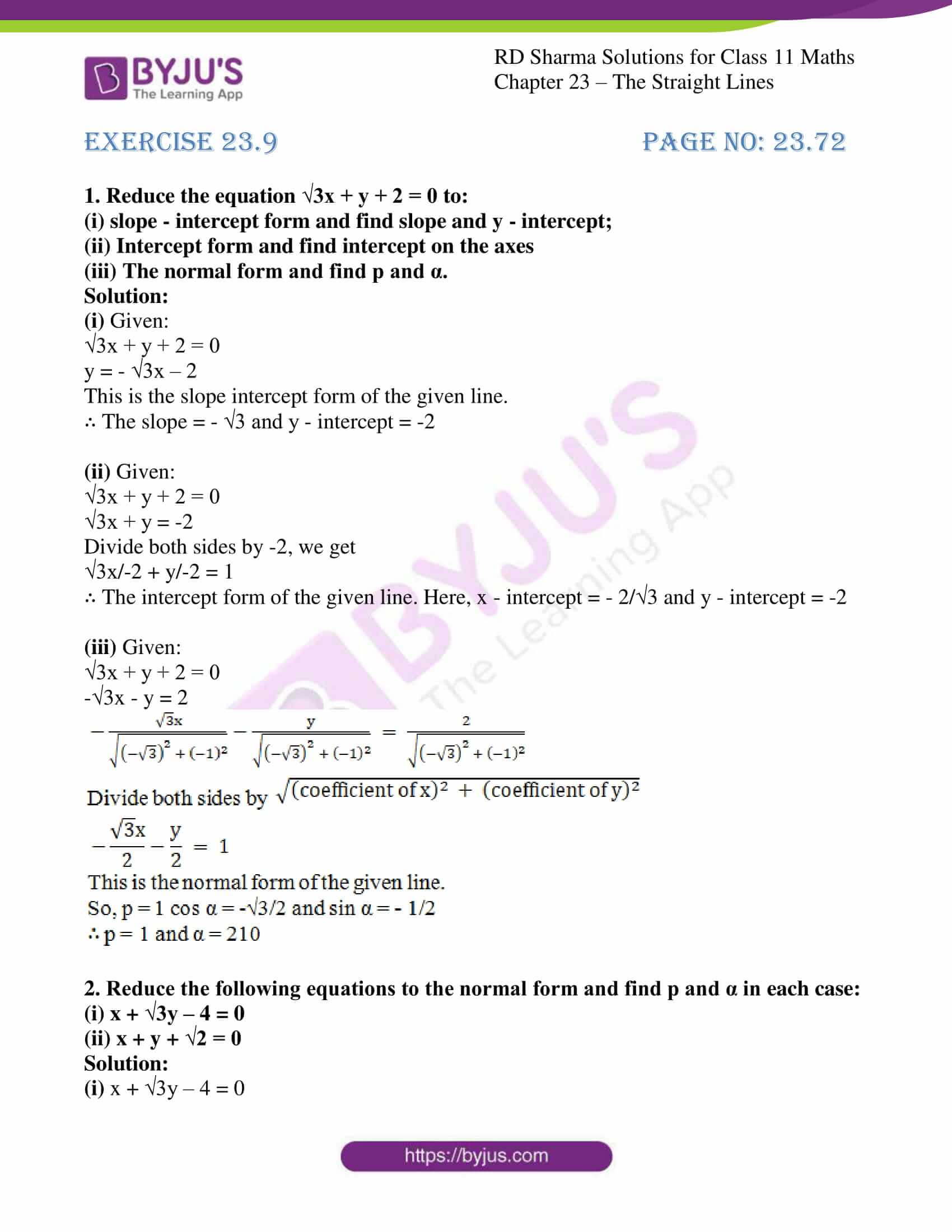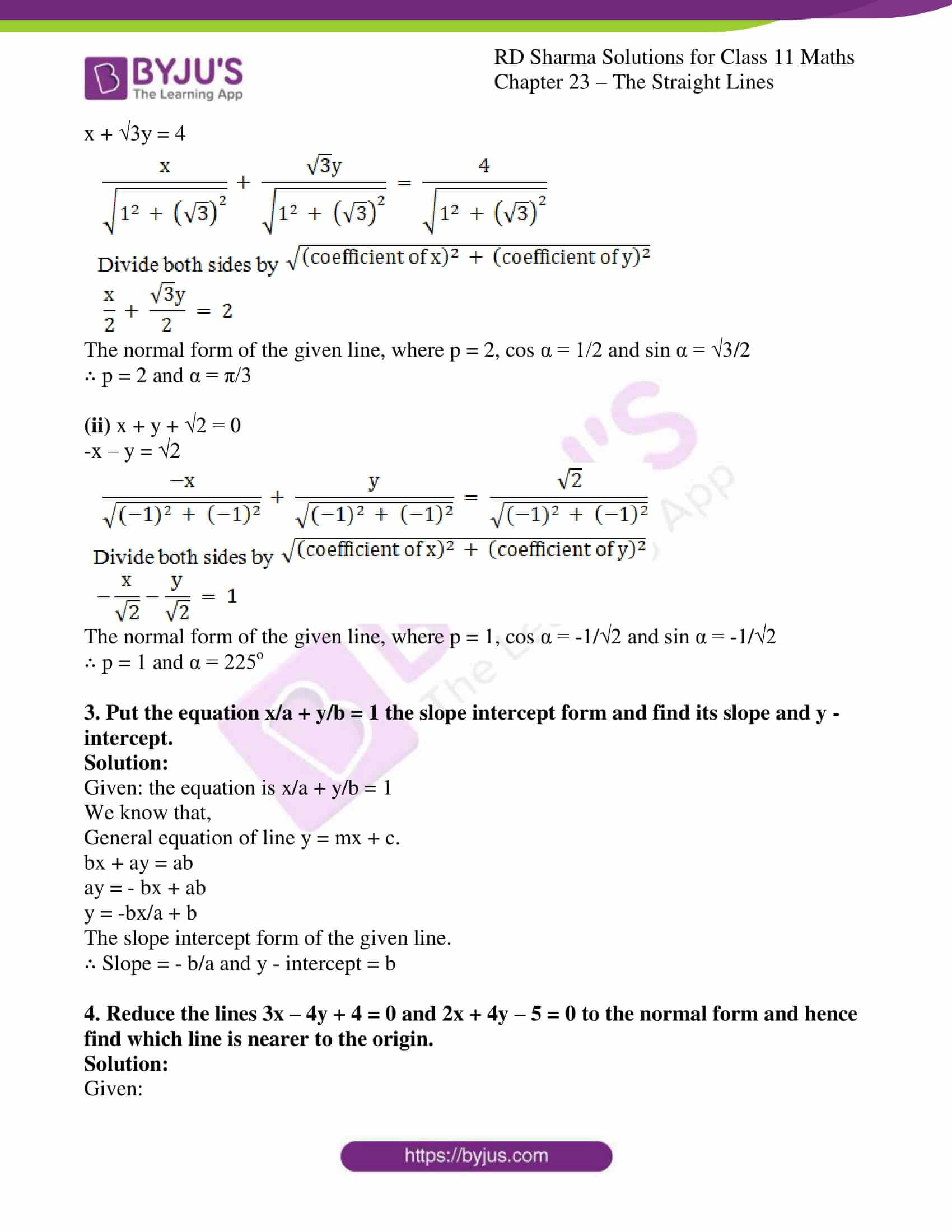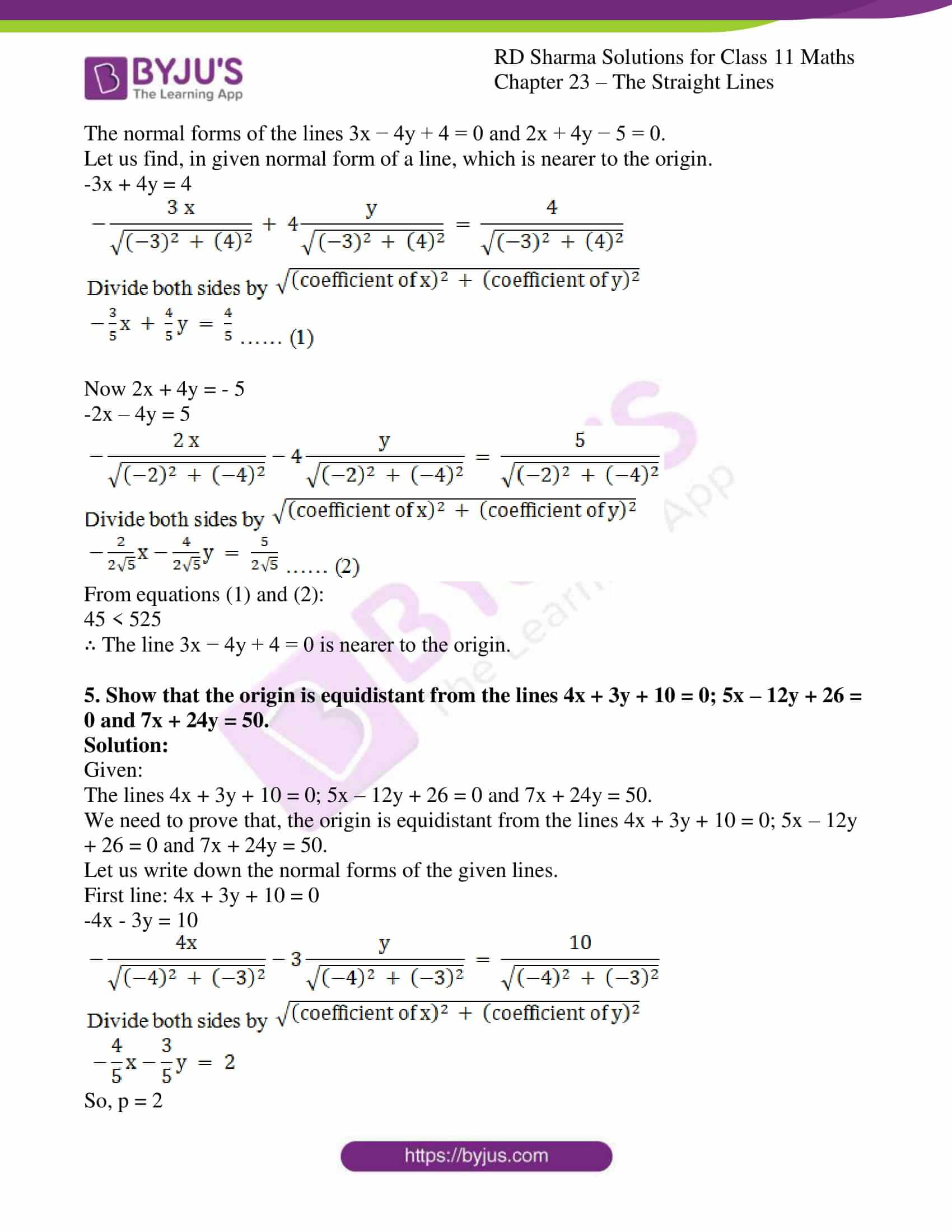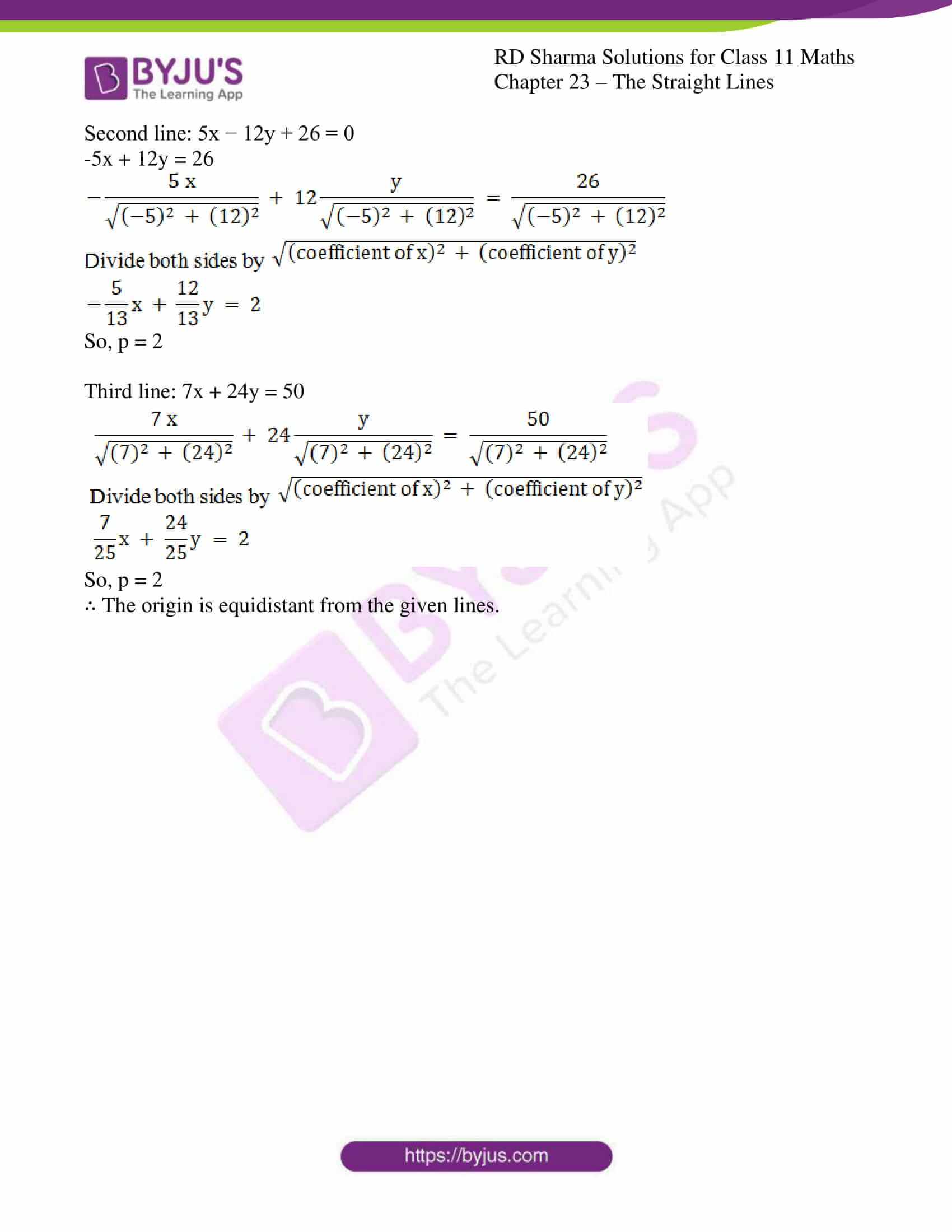### Access answers to RD Sharma Solutions for Class 11 Maths Exercise 23.9 Chapter 23 – The Straight Lines

#### EXERCISE 23.9 PAGE NO: 23.72

1. Reduce the equation √3x + y + 2 = 0 to:
(i) slope – intercept form and find slope and y – intercept;
(ii) Intercept form and find intercept on the axes
(iii) The normal form and find p and α.

Solution:

(i) Given:

√3x + y + 2 = 0

y = – √3x – 2

This is the slope intercept form of the given line.

∴ The slope = – √3 and y – intercept = -2

(ii) Given:

√3x + y + 2 = 0

√3x + y = -2

Divide both sides by -2, we get

√3x/-2 + y/-2 = 1

∴ The intercept form of the given line. Here, x – intercept = – 2/√3 and y – intercept = -2

(iii) Given:

√3x + y + 2 = 0

-√3x – y = 2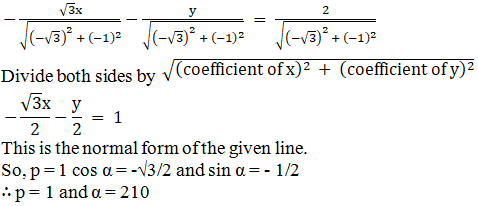2. Reduce the following equations to the normal form and find p and α in each case:
(i) x + √3y – 4 = 0
(ii) x + y + √2 = 0

Solution:

(i) x + √3y – 4 = 0

x + √3y = 4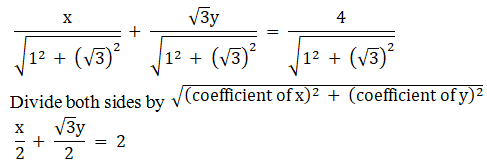The normal form of the given line, where p = 2, cos α = 1/2 and sin α = √3/2

∴ p = 2 and α = π/3

(ii) x + y + √2 = 0

-x – y = √2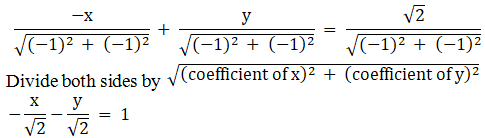The normal form of the given line, where p = 1, cos α = -1/√2 and sin α = -1/√2

∴ p = 1 and α = 225o

3. Put the equation x/a + y/b = 1 the slope intercept form and find its slope and y – intercept.

Solution:

Given: the equation is x/a + y/b = 1

We know that,

General equation of line y = mx + c.

bx + ay = ab

ay = – bx + ab

y = -bx/a + b

The slope intercept form of the given line.

∴ Slope = – b/a and y – intercept = b

4. Reduce the lines 3x – 4y + 4 = 0 and 2x + 4y – 5 = 0 to the normal form and hence find which line is nearer to the origin.

Solution:

Given:

The normal forms of the lines 3x − 4y + 4 = 0 and 2x + 4y − 5 = 0.

Let us find, in given normal form of a line, which is nearer to the origin.

-3x + 4y = 4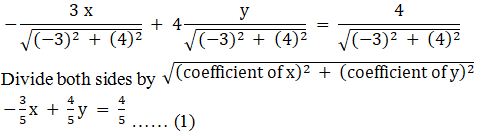Now 2x + 4y = – 5

-2x – 4y = 5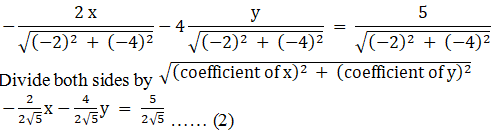From equations (1) and (2):

45 < 525

∴ The line 3x − 4y + 4 = 0 is nearer to the origin.

5. Show that the origin is equidistant from the lines 4x + 3y + 10 = 0; 5x – 12y + 26 = 0 and 7x + 24y = 50.

Solution:

Given:

The lines 4x + 3y + 10 = 0; 5x – 12y + 26 = 0 and 7x + 24y = 50.

We need to prove that, the origin is equidistant from the lines 4x + 3y + 10 = 0; 5x – 12y + 26 = 0 and 7x + 24y = 50.

Let us write down the normal forms of the given lines.

First line: 4x + 3y + 10 = 0

-4x – 3y = 10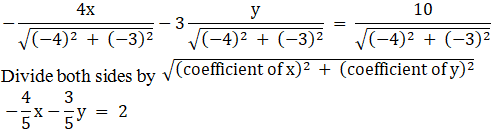So, p = 2

Second line: 5x − 12y + 26 = 0

-5x + 12y = 26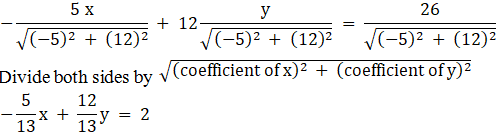So, p = 2

Third line: 7x + 24y = 50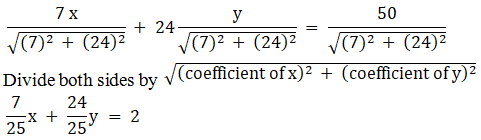So, p = 2

∴ The origin is equidistant from the given lines.